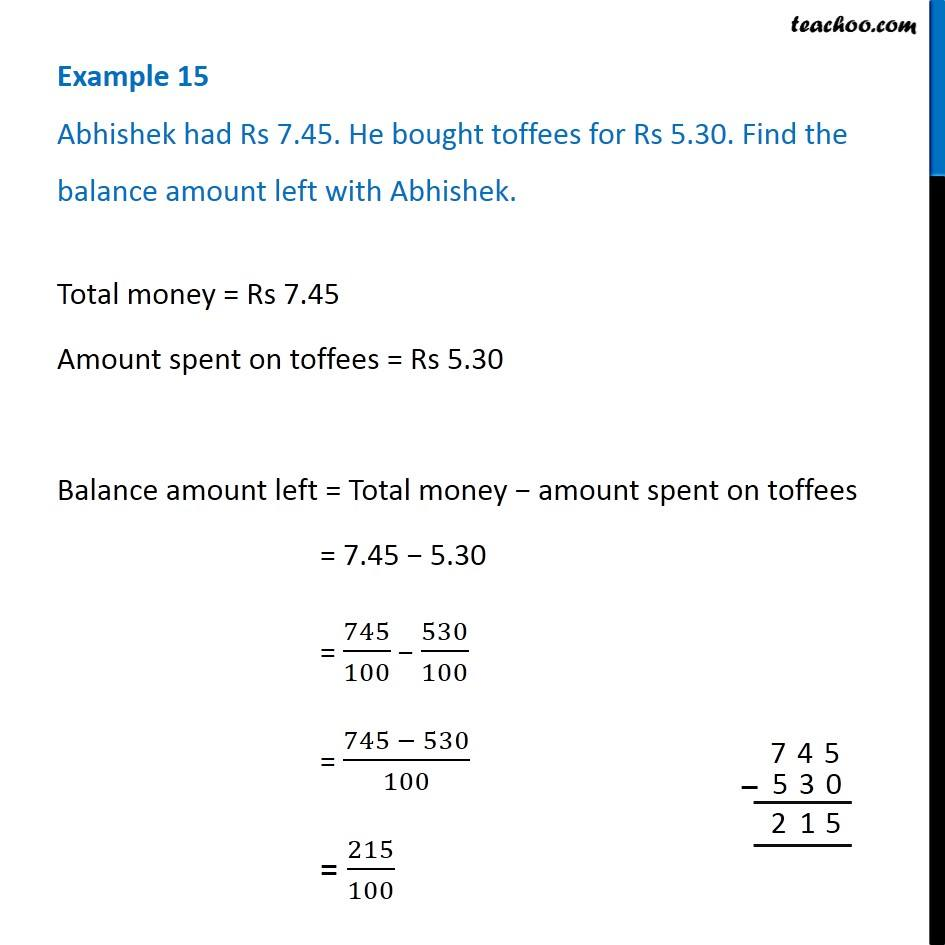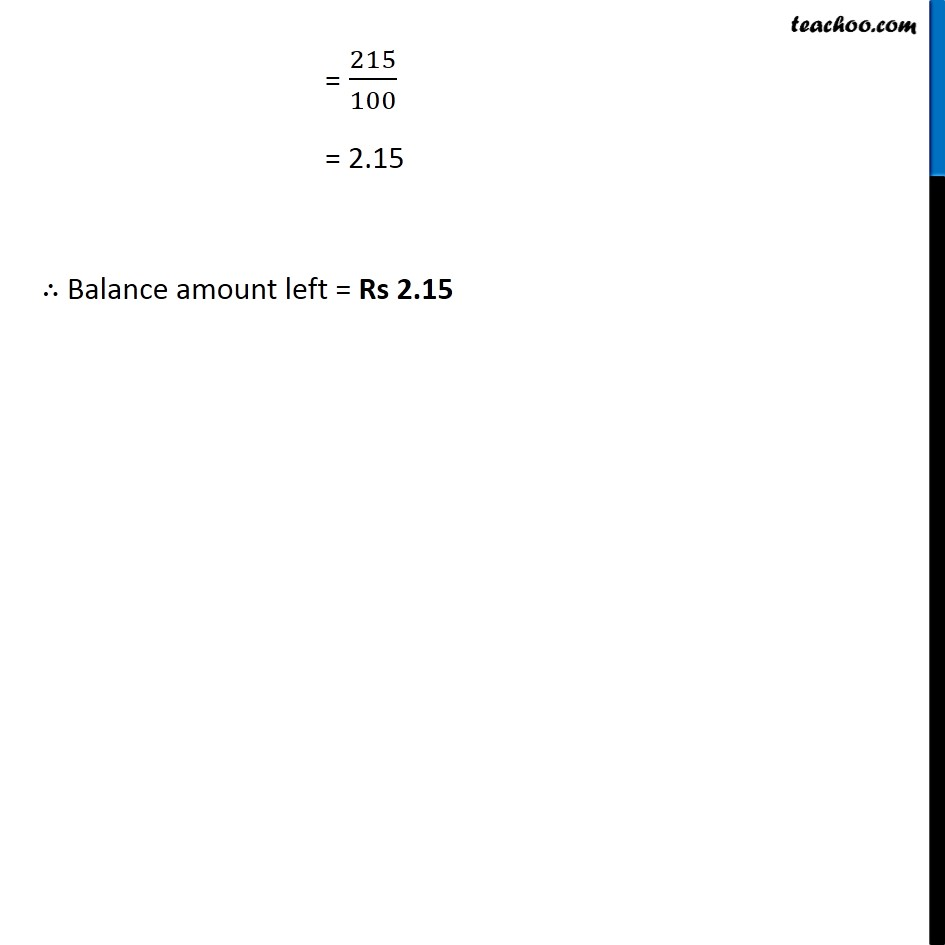Examples

Chapter 8 Class 6 Decimals
Serial order wise### Transcript

Example 15 Abhishek had Rs 7.45. He bought toffees for Rs 5.30. Find the balance amount left with Abhishek. Total money = Rs 7.45 Amount spent on toffees = Rs 5.30 Balance amount left = Total money − amount spent on toffees = 7.45 − 5.30 = 745/100 − 530/100 = (745 − 530)/100 = 215/100 = 215/100 = 2.15 ∴ Balance amount left = Rs 2.15